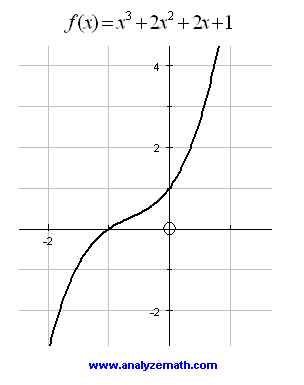# Graphs of Third Degree Polynomials

The graphs of several third degree polynomials are shown along with questions and answers at the bottom of the page.

1. Polynomial of the third degree: cuts the x axis at one point.
Question 1: Why does the graph cut the x axis at one point only?Figure 1: Graph of the third degree polynomial.

2. Polynomial of the third degree: 3 x-intercepts.
Question 2: If the graph cuts the x axis at x = -2, what are the coordinates of the two other x-intercpets?Figure 2: Graph of a third degree polynomial

3. Polynomial of the third degree: 3 x intercepts and parameter a to determine.
Question 3: The graph below cuts the x axis at x = 1 and has a y-intercpet at y = 1. What are the coordinates of the two other x intercpets?Figure 3: Graph of a third degree polynomial

4. Polynomial of a third degree polynomial: one x-intercepts.
Question 4: The graph below cuts the x axis at x = -1. Why does the graph of this polynomial have one x intercept only?Figure 4: Graph of a third degree polynomial, one intercpet.